# SBI Clerk Prelims Reasoning Ability Questions 2021 (Day-29)

Dear Aspirants, Our IBPS Guide team is providing new series of Reasoning Questions for SBI Clerk Prelims 2021 so the aspirants can practice it on a daily basis. These questions are framed by our skilled experts after understanding your needs thoroughly. Aspirants can practice these new series questions daily to familiarize with the exact exam pattern and make your preparation effective.

Start Quiz

Floor based puzzle

Direction (1-5): Study the following information carefully and answer the below questions

Eight persons- A to H are living in the eight-storey building. The lowermost floor is numbered as one and the topmost floor is numbered as eight. All the information is not necessarily in the same order.

F lives on an even number floor two persons above G. Number of person lives above F is the same as below B. H lives immediately above the one who lives two persons above A. H is neither living adjacent floor of G nor E. E lives below D and is not living on the lowermost floor.

1) How many floors are between H and A?

A.As many as between F and B.

B.Three

C.As many as between D and A.

D.Four

E.None of the above

2) Which of the following statement is/are true?

I). Four floors between H and B.

II). E lives two floors above C.

III). F lives immediately above A.

A.Only I and III

B.Only III

C.Only I and II

D.Only II

E.None of the statement is false

3) As many people live above D is same as that of below___?

A.G

B.A

C.F

D.E

E.C

4) Who among the following lives three floors above B?

A.The one who lives on the sixth floor

B.The one who lives immediately above D

C.The one who lives two floors below H.

D.No one

E.Both a and c

5) What is the position of G from the top of the building?

A.Seventh

B.Fourth

C.Sixth

D.Fifth

E.None of the above

Direction sense

Direction (6-8): Study the following information carefully and answer the below questions

Point P is 2m north of Point Q. Point Z is 9m west of Point Q. Point L is 15m east of Point M which is 20m south of Point Z. Point R is 3m south of Point L. Point T is west of point R and South of Point P.

6) How far and what is the direction of Q with respect to T?

A.21m towards the south

B.20m towards the south

C.23m towards the north

D.22m towards the north

E.None of the above

7) What is the shortest distance between Point L and Point Z?

A.21m

B.24m

C.20m

D.23m

E.25m

8) What is the shortest distance between T and R?

A.6m

B.15m

C.7m

D.9m

E.None of the above

Inequality

Direction (9-10): In these questions, the relationship between different elements is shown in the statements. These statements are followed by two conclusions. Find the conclusion which logically follows.

(a) If only conclusion I follow.

(b) If only conclusion II follows.

(c) If either conclusion I or II follows.

(d) If neither conclusion I nor II follows.

(e) If both conclusion I and II follow.

9) Statements:

P ≥ N = G > T < K > L

Conclusion:

I). G < K

II). P > T

10) Statements:

Q > K > L ≤ C = H > I

Conclusion:

I). K > I

II). H > L

Directions (1-5):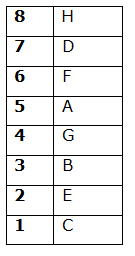F lives on an even number floor two persons above G.

Number of person lives above F is same as below B.

From the above condition, there are three possibilities.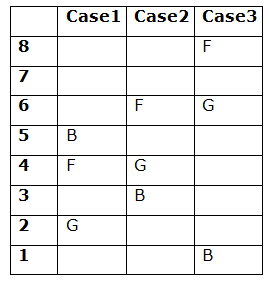H lives immediately above the one who lives two persons above A.

H is neither living adjacent floor of G nor E.

From the above condition, case3 gets eliminated.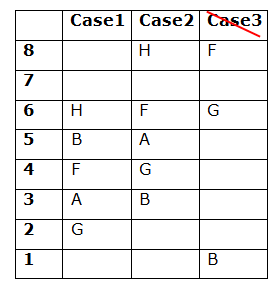E lives below D and is not living on the lowermost floor.

From the above condition, case1 gets eliminated. Case2 shows the final arrangement.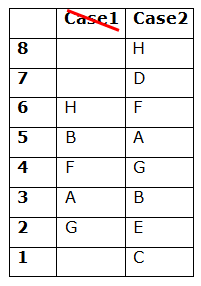Directions (6-8):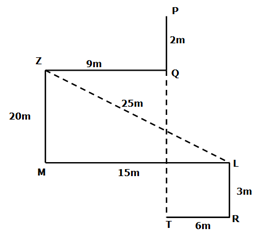202+152=ZL2

√(202+152)=√625=25m